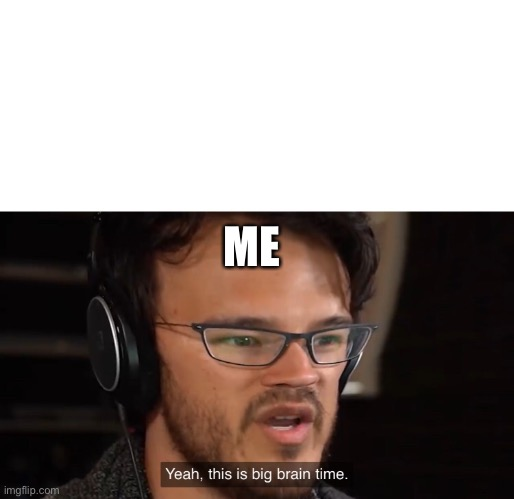Meme_Maker_Masta (38690)
Joined 2019-10-25
(try to find the "e" FFFFFFFFFFFFFFFFFFFFFFFFFFFFFFFFFFFFFFFFFFFFFFFFFEFFFFFFFFFFFFFFFFFFFFFFFFFFFFFFFFFFFFFFFFFFFFFFFFF
62 Featured Images
168 Creations
3 Followers

See what thsi mean i will do suprize ._. in fun
0 ups, 1w
You said "Nani" wrong and it means what.
infinite confusion in fun